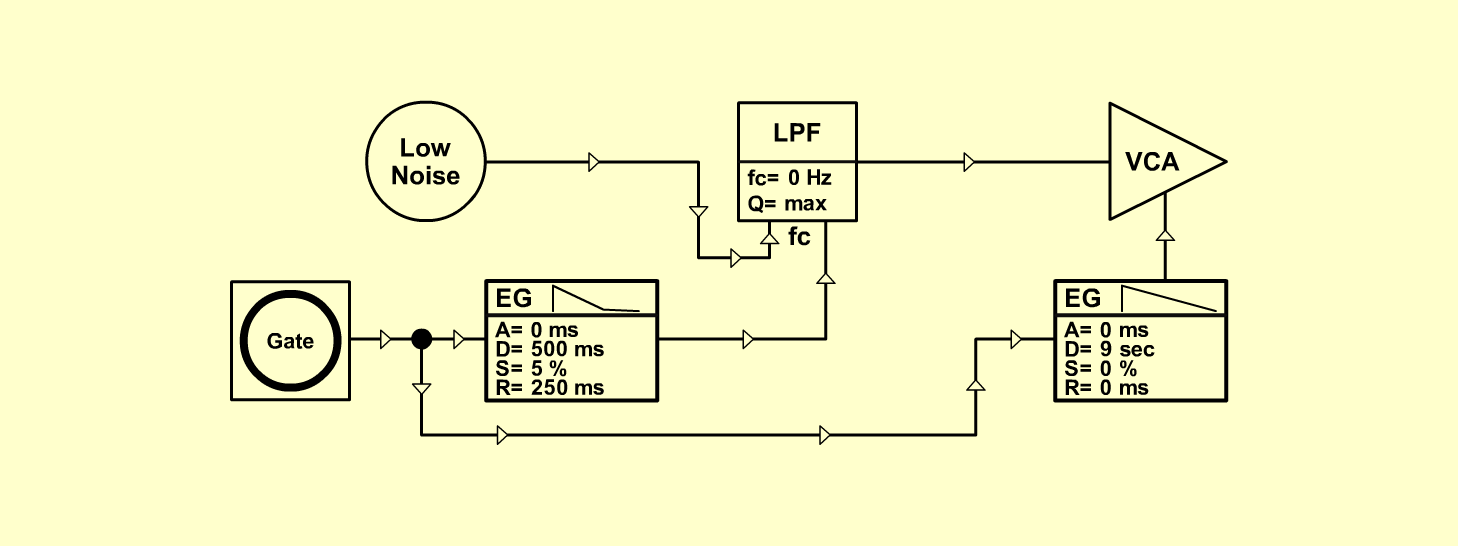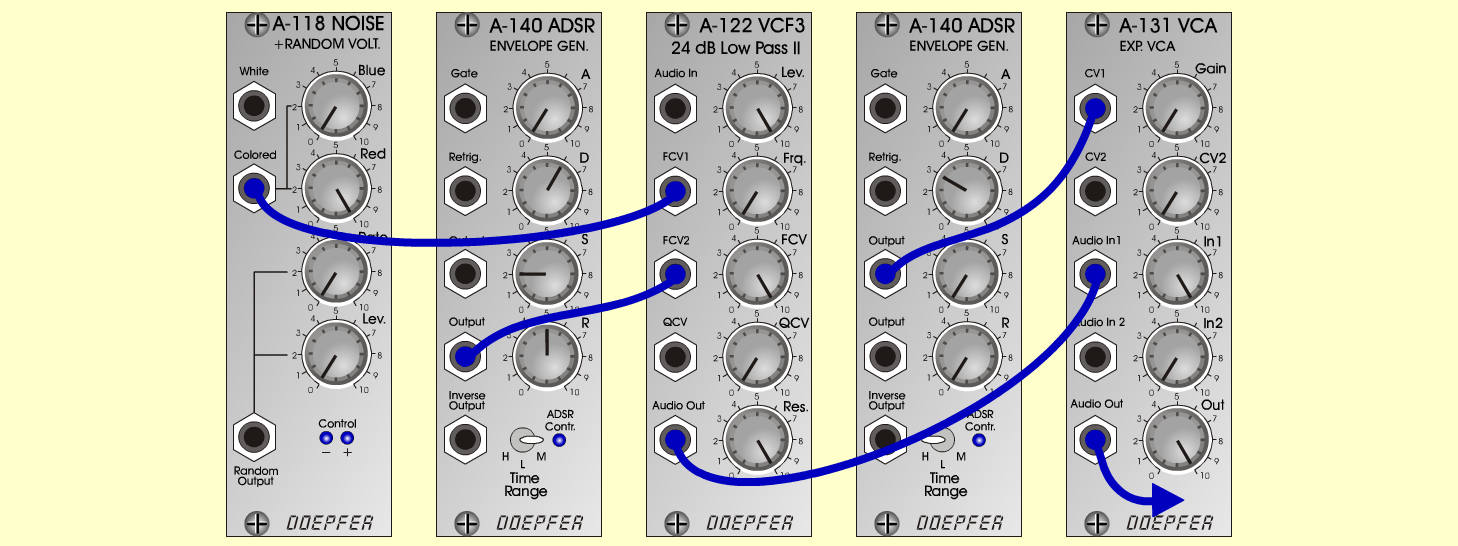SupernovaFXD o e p f e r A - 100 Connections: Settings: A-118 (Colored)  <=>  A-122 (FCV 1) A-140/1 (Output)  <=>  A-122 (FCV 2) A-122 (Audio Out)  <=>  A-131 (Audio In) A-140/2 (Output)  <=>  A-131 (CV 1) A-131 (Output)  <=>  Amplifier A-118 (Blue = 0, Red = 10, Rate = 0, Level = 0) A-122 (Level = 10, Freq = 0, FCV  = 10, Res = 10) A-140/1 (A = 0, D = 6, S = 2, R = 5, Range = M) A-140/2 (A = 0, D = 3, S = 0, R = 0, Range = H) A-131 (Gain = 0, Audio In 1 = 10,  Audio Out = 10) Josef MuellerSound sample Supernova